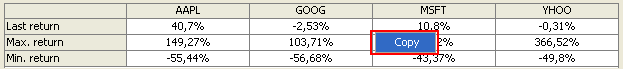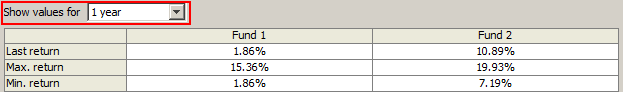# Tables

## What can we see in the tables?

A table is shown in both comparison and single view.

• In single view, the table shows the yield of the last period, average, the worst and the best yield, and standard deviation for each period.• In comparison view, the yield of the last period, the worst and the best yield are shown for all loaded investments.In relation to calculations it must be noted, that

• Values given in both tables are calculated from the entire time-range.

• Values given for periods shorter than one year are nominal, those past a year are calculated per annum.

The risk has several assessment numbers. One of them is the standard deviation, which is calculated from the difference of the return from the average return. The smaller the difference from the average return is, the smaller is the standard deviation and the risk as well. (When comparing the standard deviations it is important to remember that the standard deviation of every security must be calculated based on the same amount of data!)

Why is the standard deviation important? As the return is with 95% probability within the standard deviation band of +/-2 around the average return. And it is not at all irrelevant how wide is the band, within which the return can change, is it? (If, for example, the average return is 15% and the standard deviation is 20%, then the return will be between 15-2*20 = -25% and 15%+2*20% = 55% with a probability rate of 95%.

• For the calculation of standard deviation, at least 2 periods are required (for one month’s deviation 2 months, for one year’s deviation 2 years, and so on). This is why in case of having for example: 8 years of data, a five-year standard deviation cannot be calculated.

## What are these tables useful for?

When searching for the top performer investment you mainly find rankings, which were prepared based on the returns of the last 1 year. As if nothing else would matter!

In reality, the following questions are absolutely just:

• Why should you choose the one, which was the top performer in the last 1 year?
• What if the good return of the last 1 year was just the result of a special luck?
• What if the fund showed no good performance before and will not perform in the future either?
• What if another fund will easily outrun it?

Beside the last 1 year return there are some other values, which are worth to examine:

• If the average return was high back for a long period of time, that is probably not the matter of luck.
• You make no harm either if you consider the best and the worst performance in a 1 year period of time and prepare for the best and the worst cases.

## How can the tables be used?

Contents of tables can be copied to the clipboard (and pasted into, for example a Microsoft Excel file). To do so:

• Right click the given table.

• From the pop-up menu select “Copy”.In comparison view, the time-range for displaying data of investments can be set in a drop-down menu: